# Finding Square Root by Division Method:

When the numbers are large, even the method of finding square root by prime factorization becomes lengthy and difficult. To overcome this problem we use Long Division Method.

Consider the following steps to find the square root of 529.

Step 1: Place a bar over every pair of digits starting from the digit at one’s place. If the number of digits in it is odd, then the left-most single-digit too will have a bar. Thus we have, bar(5) bar(29).

Step 2: Find the largest number whose square is less than or equal to the number under the extreme left bar (22 < 5 < 32). Take this number as the divisor and the quotient with the number under the extreme left bar as the dividend (here 5).
Divide and get the remainder (1 in this case).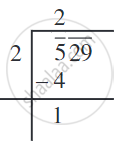Step 3: Bring down the number under the next bar (i.e., 29 in this case) to the right of the remainder. So the new dividend is 129.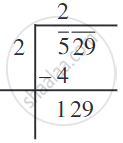Step 4: Double the quotient and enter it with a blank on its right.

Step 5: Guess the largest possible digit to fill the blank which will also become the new digit in the quotient, such that when the new divisor is multiplied to the new quotient the product is less than or equal to the dividend.
In this case 42 × 2 = 84.
As 43 × 3 = 129 so we choose the new digit as 3. Get the remainder.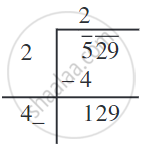Step 6: Since the remainder is 0 and no digits are left in the given number, therefore, sqrt(529) = 23.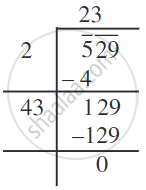Now consider sqrt(4096).

Step 1: Place a bar over every pair of digits starting from the one’s digit. (bar(40)  bar(96)).

Step 2: Find the largest number whose square is less than or equal to the number under the left-most bar (62 < 40 < 72). Take this number as the divisor and the number under the leftmost bar as the dividend.
Divide and get the remainder i.e., 4 in this case.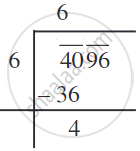Step 3: Bring down the number under the next bar (i.e., 96) to the right of the remainder. The new dividend is 496.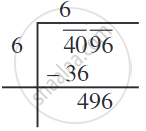Step 4: Double the quotient and enter it with a blank on its right.

Step 5: Guess the largest possible digit to fill the blank which also becomes the new digit in the quotient such that when the new digit is multiplied to the new quotient the product is less than or equal to the dividend. In this case, we see that 124 × 4 = 496.
So the new digit in the quotient is 4. Get the remainder.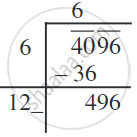Step 6: Since the remainder is 0 and no bar left, therefore, sqrt(4096) = 64.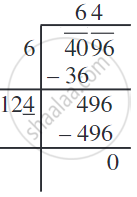#### Example

Find the square root of 1296.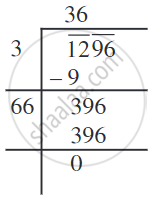Therefore, sqrt(1296) = 36.

#### Example

Find the greatest 4-digit number which is a perfect square.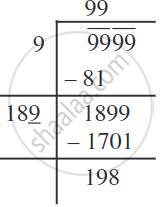Greatest number of 4-digits = 9999.
We find sqrt(9999) by long division method. The remainder is 198.
This shows 992 is less than 9999 by 198.
This means if we subtract the remainder from the number, we get a perfect square.
Therefore, the required perfect square is 9999 - 198 = 9801.
And, sqrt(9801) = 99.
If you would like to contribute notes or other learning material, please submit them using the button below.

### Shaalaa.com

Finding Square Root by Long Division Method [00:11:43]
S
0%